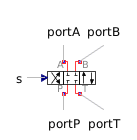Pressure Sensor - MapleSim Help

Pressure Sensor

Sense pressure at port with respect to the provided pressureDescription The Pressure Sensor component generates an output signal of the pressure at the port with respect to the Reference pressure.Equations $y=\mathrm{toUnit}\left({p}_{\mathrm{port}}-{P}_{0}\right)$ $\mathrm{mflow}=0\phantom{\rule[-0.0ex]{0.0em}{0.0ex}}\phantom{\rule[-0.0ex]{0.0em}{0.0ex}}$Connections

 Name Description Modelica ID $\mathrm{port}$ Pneumatic port port $y$ Real output signal in selected units yParameters

 Name Default Units Description Modelica ID ${P}_{0}$ $0$ $\mathrm{Pa}$ Reference pressure P0 To unit $\mathrm{Pa}$ Output unit toUnit